Courses
Courses for Kids
Free study material
Free LIVE classes
More

# NCERT Solutions for Class 10 Maths Chapter 11 Constructions (Ex 11.2) Exercise 11.2## NCERT Solutions for Class 10 Maths Chapter 11 Constructions (Ex 11.2) Exercise 11.2

Last updated date: 02nd Jun 2023
Total views: 477.9k
Views today: 13.33k

## Access NCERT Solutions for Class 10 Maths Chapter 11 – Constructions

Exercise No: 11.2

1. Draw a circle of radius $6$ cm. From a point $10$ cm away from its centre, construct the pair of tangents to the circle and measure their lengths. Give the justification of the construction.

Ans: A pair of tangents to the given circle can be constructed as follows.

Step 1. Draw a circle with the centre as $O$ and its radius is $6$ cm.

Step 2. Mark a point $10$ cm away from $O$ and name it as $P$. Now, join the points $OP$.

Step 3. Draw the perpendicular bisector on $OP$ and name the mid-point as $M$.

Step 4. Taking $M$ as a centre and $MO$ as a radius, draw a circle.

Step 5. And new circle intersects the previous circle at points $Q$ and $R$.

Step 6. Now join $PQ$ and $PR$. Then the required tangents are $PQ$ and $PR$.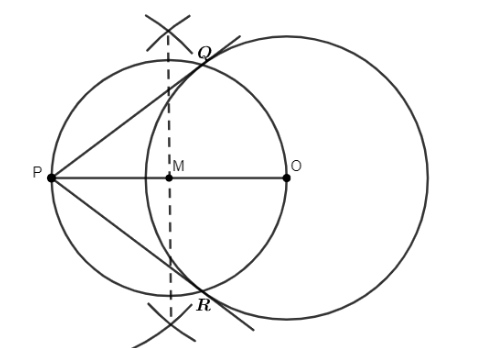The lengths of tangents $PQ$ and $PR$ are $8$ cm each.

Justification

The construction can be justified by proving that $PQ$ and $PR$ are the tangents to the circle, whose centre is $O$ and its radius is $6$ cm.

Now, join $OQ$ and $OR$.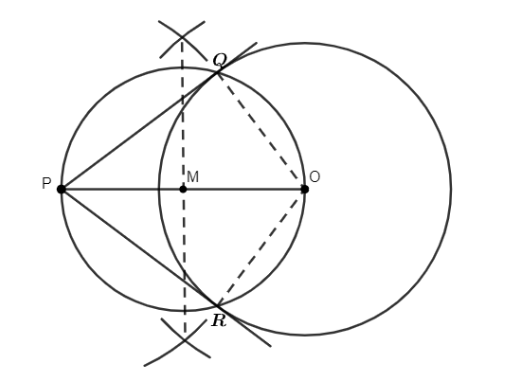Now in triangle $PQO$,

$\angle PQO=90{}^\circ$

Then, by using Pythagoras’ theorem.

$PQ=\sqrt{O{{P}^{2}}-O{{Q}^{2}}}$

$=\sqrt{{{\left( 10 \right)}^{2}}-{{6}^{2}}}$

$=\sqrt{100-36}$

$=\sqrt{64}$

$=8$ cm.

Similarly, $PR=8$cm.

$\therefore OQ\bot PQ$.

Since $OQ$ is the radius of the circle, $PQ$ has to be a tangent of the circle.

Similarly, $PR$ is a tangent of the circle.

2. Construct a tangent to a circle of radius $4$ cm from a point on the concentric circle of radius $6$ cm and measure its length. Also verify the measurement by actual calculation. Give the justification of the construction.

Ans: Tangent on the given circle can be drawn as follows.

Step 1. Draw a circle with the centre as $O$ and its radius is $4$ cm.

Step 2. Again, draw a circle with the centre as $O$ and its radius is $6$ cm. Locate a point $P$ on this circle and join $OP$.

Step 3. Draw a bisector on $OP$. And $M$be the midpoint of $OP$.

Step 4. Taking $M$ as the centre and $MO$ as its radius, draw a circle. And this circle intersects at the previous circle at the points $Q$ and $R$.

Step 5. Now, join $PQ$ and $PR$. These are the required tangents.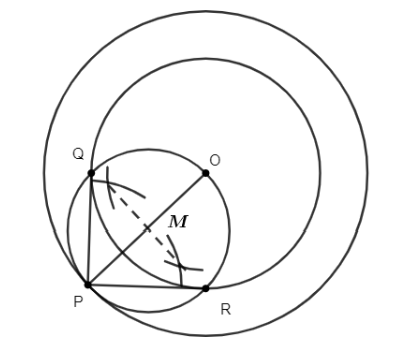Let us find the length of $PQ$ and $PR$. Then,

In $\Delta PQO$,

Since $PQ$ is a tangent,

$\angle PQO=90{}^\circ$

$PO=6$ cm and $QO=4$ cm

By using Pythagoras theorem, we get

$P{{Q}^{2}}=P{{O}^{2}}-Q{{O}^{2}}$

$={{6}^{2}}-{{4}^{2}}$

$=36-16=20$

$\Rightarrow PQ=\sqrt{20}=4.47$

$\therefore PQ=4.47$ cm

Similarly, $PR=4.47$ cm

Therefore, the length of each tangent is $4.47$ cm.

Justification:

The construction can be justified by proving that $PQ$ and $PR$ are the tangents of the circle and whose centre is $O$ and radius is $4$ cm. Now, let us join $OQ$ and $OR$.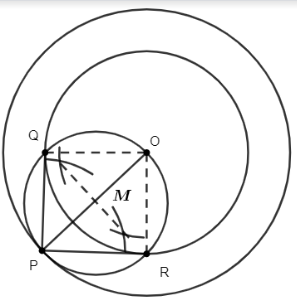$\angle PQO$ is an angle in the semi-circle. And the angle in a semicircle is a right angle.

$\therefore \angle PQO=90{}^\circ$

We know that $OQ\bot PQ$.

Since $OQ$ is the radius of the circle, then $PQ$ has to be a tangent of the circle. Similarly, $PR$ is also a tangent of the circle.

Hence proved.

3. Draw a circle of radius $3$ cm. Take two points $P$ and $Q$ on one of its extended diameters each at a distance of $7$ cm from its centre. Draw tangents to the circle from these two points $P$ and $Q$. Give the justification of the construction.

Ans: The tangent can be constructed on the given circle as follows.

Step 1. Draw a circle with the centre as $O$ and its radius is $3$ cm.

Step 2. Now, take one of its diameters, $PQ$ and extend it on both sides. Then, locate two points on this diameter such that $OR=OS=7$ cm.

Step 3. Draw a bisector on the $OR$ and similarly on the $OS$. Let $T$ and $U$be the mid-points of $OR$ and $OS$.

Step 4. Taking $T$ and $U$ as its centre, then with $TO$ and $UO$ as radius, draw two circles. These two circles will intersect at the previous circle at points $V,W,X,$ and $Y$ respectively.

Step 5. Now, join $RV,RW,SX,$ and $SY$. Then, these are the required tangents.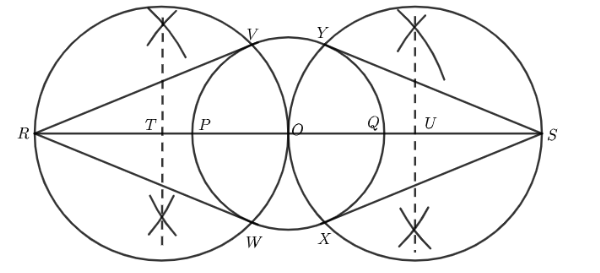Justification

The construction can be justified by proving that $RV,RW,SX,$ and $SY$ are the tangents to the circle and whose centre is $O$, then its radius is $3$ cm. Now, let us join $OV,OW,OX,$ and $OY$.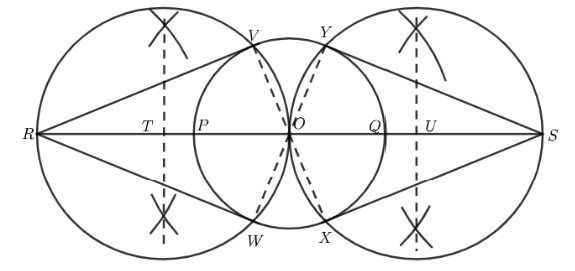$\angle RVO$ is an angle in the semi-circle. And the angle in a semicircle is a right angle.

$\therefore \angle RVO=90{}^\circ$.

We know that $OV\bot RV$.

Since $OV$ is the radius of the circle, $RV$ has to be a tangent of the circle. Similarly, $OW,OX,$ and $OY$ are the tangents of the circle.

Hence proved.

4. Draw a pair of tangents to a circle of radius $5$ cm which are inclined to each other at an angle of $60{}^\circ$. Give the justification of the construction.

Ans: The tangents can be constructed as follows.

Step 1. Draw a circle with the centre as $O$ and its radius is $5$ cm.

Step 2. Take a point $A$ on the circumference of the circle and join $OA$. Draw a perpendicular to $OA$ at point $A$.

Step 3. Draw a radius of $OB$, making an angle of $120{}^\circ$ with $OA$.

Step 4. Draw a perpendicular line to $OB$ at point $B$. Let both the perpendiculars intersect at a point $P$.

Step 5. Then, $PA$ and $PB$ are the required tangents at an angle of  $60{}^\circ$.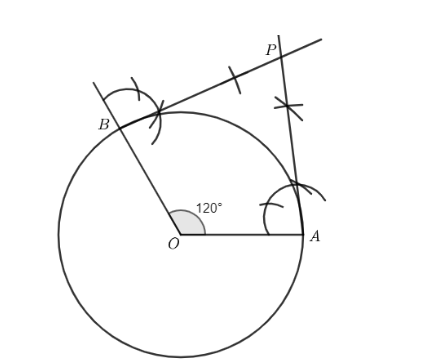Justification

The construction can be justified by proving that $\angle APB=60{}^\circ$

By our construction

$\angle OAP=90{}^\circ$, $\angle OBP=90{}^\circ$ and $\angle AOB=120{}^\circ$.

We know that the sum of all interior angles of a quadrilateral is $360{}^\circ$

Then, $\angle OAP+\angle AOB+\angle OBP+\angle APB=360{}^\circ$

$90{}^\circ +120{}^\circ +90{}^\circ +\angle APB=360{}^\circ$

$300{}^\circ +\angle APB=360{}^\circ$

$\angle APB=60{}^\circ$

Hence proved.

5. Draw a line segment $AB$ of length $8$ cm. Taking $A$ as centre, draw a circle of radius $4$ cm and taking $B$ as centre, draw another circle of radius $3$ cm. Construct tangents to each circle from the centre of the other circle. Give the justification of the construction.

Ans: The tangents can be constructed on the given circles as follows.

Step 1. Draw a line segment $AB$ of $8$cm. Taking $A$ and $B$ as centre, draw two circles of radii are $4$cm and $3$ cm.

Step 2. Draw the bisector on the line $AB$. Let $C$ be the midpoint of $AB$.

Step 3. Taking $C$ as centre, draw a circle of radius as $AC$. Then, it intersects the circles at points of $P,Q,R,$ and $S$.

Step 4. Now, join $BP,BQ,AS,$ and $AR$. Then, these are the required tangents.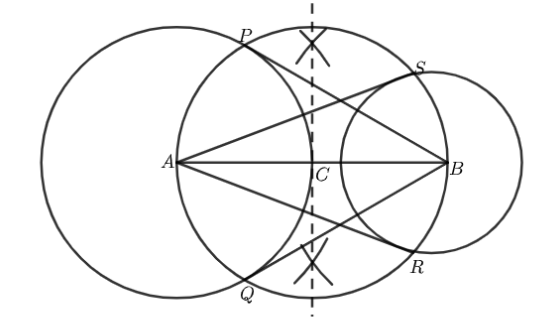Justification

The construction can be justified by proving that $AS$ and $AR$ are the tangents of the circle and whose centre is $B$ and radius is $3$ cm and $BP$ and $BQ$ are the tangents of the circle and whose centre is $A$ and radius is $4$ cm. For this, join $AP,\text{ }AQ,\text{ }BS,$and $BR$.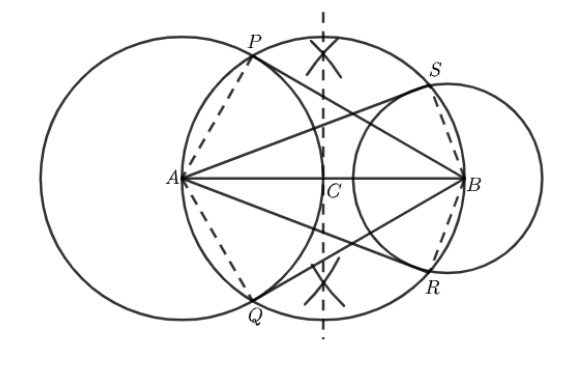$\angle ASB$is an angle in the semi-circle. We know that an angle in a semicircle is a right angle.

$\therefore \angle ASB=90{}^\circ$

$\Rightarrow BS\bot AS$

Since $BS$ is the radius of the circle, $AS$ has to be a tangent of the circle. Similarly, $AR,\text{ }BP,$and $BQ$ are the tangents.

Hence proved.

6. Let $\mathbf{ABC}$ be a right triangle in which $\mathbf{AB}\text{ }=\text{ }\mathbf{6}\text{ }\mathbf{cm},\text{ }\mathbf{BC}\text{ }=\text{ }\mathbf{8}\text{ }\mathbf{cm}$ and $\angle \mathbf{B}\text{ }=\text{ }\mathbf{90}{}^\circ .\text{ }\mathbf{BD}$ is the perpendicular from $\mathbf{B}$ on $\mathbf{AC}$. The circle through $\mathbf{B},\text{ }\mathbf{C},$ and $\mathbf{D}$ is drawn. Construct the tangents from $\mathbf{A}$ to this circle. Give the justification of the construction.

Ans: Consider the case below. If you draw a circle across $B,\text{ }D,$ and $C$, the diameter will be $BC$ because $BDC$ is $90{}^\circ$. The midpoint of $BC$ will be the centre $E$ of this circle.On the provided circle, the needed tangents can be created as follows.

Step 1. Join $AE$ and cut it in half. Let $F$ be the midpoint of $AE$.

Step 2. Draw a circle with $F$ as the centre and $FE$ as the radius, intersecting the circle at points $B$ and $G$. Participate in $AG$.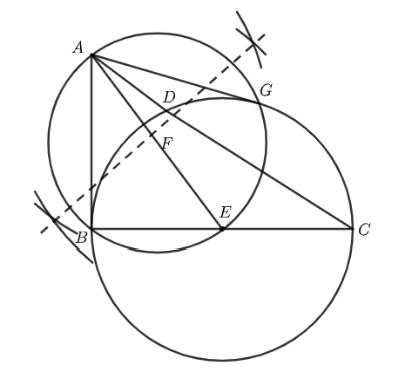The required tangents are $AB$ and $AG$.

Justification

The structure can be justified by demonstrating that $AG$ and $AB$ are the circle's tangents. Join $EG$ for this.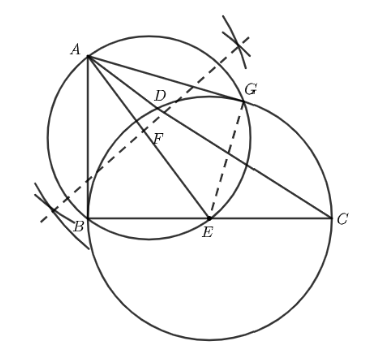$\angle AGE$ is an angle in the semi-circle. We know that an angle in a semicircle is a right angle.

$\therefore \angle AGE=90{}^\circ$

$\Rightarrow EG\bot AG$

Since $EG$ is the radius of the circle, $AG$ has to be a tangent of the circle.

$\Rightarrow AB\bot BE$

Since $BE$ is the radius of the circle, $AB$ has to be a tangent of the circle.

Hence proved.

7. Draw a circle with the help of a bangle. Take a point outside the circle. Construct the pair of tangents from this point to the circles. Give the justification of the construction.

Ans: On the provided circle, the needed tangents can be created as follows. Step 1. With the help of a bangle, draw a circle.

Step 2. Draw two chords $QR$ and $\text{ }ST$ from a point $P$ outside the circle.

Step 3. Draw perpendicular bisectors of these chords and allow them to connect at point $O$.

Step 4. Join $PO$ and cut it in half. Let $U$ be the midpoint of $PO$. Draw a circle

with a radius of $OU$ around $U$, intersecting the circle at $V$ and $W$. $PV$and

$PW$ should team together.

The required tangents are $PV$ and $PW$.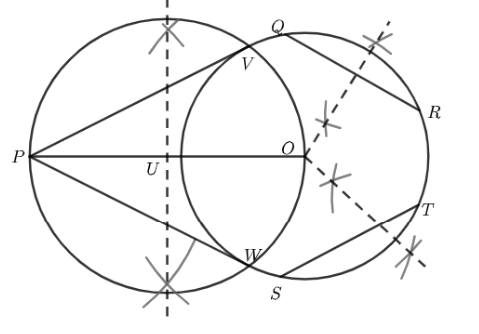Justification

The structure can be justified by demonstrating that $PV$ and $PW$ are the circle's tangents. To do so, it must first be established that $O$ is the circle's centre. Let's join forces with $OV$ and $OW$.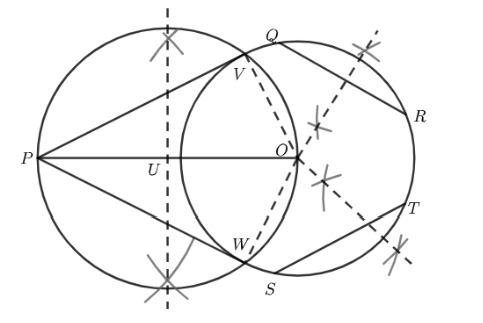The perpendicular bisector of a chord is known to travel through its centre.

As a result, the centre is bisected by the perpendicular bisector of chords $QR$ and $ST$.

The junction point of these perpendicular bisectors is clearly the circle's centre. $\angle PVO$ denotes a semi-circular angle. A right angle is defined as an angle in a semicircle.

$\therefore \angle PVO=90{}^\circ$

$\Rightarrow OV\bot PV$

$PV$ must be a tangent of the circle since $OV$ is the radius of the circle. $PW$ is also a tangent to the circle.

Hence proved.

### NCERT Solutions for Class 10 Maths PDFs (Chapter-wise)

Along with this, students can also download additional study materials provided by Vedantu, for Chapter 10 of CBSE Class 10 Maths Solutions –

### Points Discussed in Exercise 11.2

Exercise 11.2 is based on the construction of a tangent on a circle from a point that lies outside the circle. Earlier, we have learnt that we cannot draw the tangent of a circle from a point that lies inside the circle. Also, we can draw only one tangent from the point that lies on the circle.

## NCERT Solutions for Class 10 Maths Chapter 11 Constructions Exercise 11.2

Opting for the NCERT solutions for Ex 11.2 Class 10 Maths is considered as the best option for the CBSE students when it comes to exam preparation. This chapter consists of many exercises. Out of which we have provided the Exercise 11.2 Class 10 Maths NCERT solutions on this page in PDF format. You can download this solution as per your convenience or you can study it directly from our website/ app online.

Vedantu in-house subject matter experts have solved the problems/ questions from the exercise with the utmost care and by following all the guidelines by CBSE. Class 10 students who are thorough with all the concepts from the Subject Maths textbook and quite well-versed with all the problems from the exercises given in it, then any student can easily score the highest possible marks in the final exam. With the help of this Class 10 Maths Chapter Constructions Exercise 11.2 solutions, students can easily understand the pattern of questions that can be asked in the exam from this chapter and also learn the marks weightage of the chapter. So that they can prepare themselves accordingly for the final exam.

Besides these NCERT solutions for Class 10 Maths Chapter Constructions Exercise 11.2, there are plenty of exercises in this chapter which contain innumerable questions as well. All these questions are solved/answered by our in-house subject experts as mentioned earlier. Hence all of these are bound to be of superior quality and anyone can refer to these during the time of exam preparation. In order to score the best possible marks in the class, it is really important to understand all the concepts of the textbooks and solve the problems from the exercises given next to it.

Do not delay any more. Download the NCERT solutions for Class 10 Maths Chapter Constructions Exercise 11.2 from Vedantu website now for better exam preparation. If you have the Vedantu app in your phone, you can download the same through the app as well. The best part of these solutions is these can be accessed both online and offline as well.

## FAQs on NCERT Solutions for Class 10 Maths Chapter 11 Constructions (Ex 11.2) Exercise 11.2

1. How many sums are there in the NCERT Solutions for Class 10 Maths Chapter 11 Constructions (Ex.-11.2) Exercise 11.2?

There are 7 sums in the NCERT Solutions for Class 10 Maths Chapter 11 Constructions (Ex.-11.2) Exercise 11.2. This chapter consists of two exercises and the first exercise Ex.-11.1 also consists of 7 sums. All these sums are solved in the NCERT Solutions PDF on Vedantu.

2. What are the main construction topics covered in the NCERT Solutions for Class 10 Maths Chapter 11 Constructions (Ex.-11.2) Exercise 11.2?

The main topics for construction that are covered in the NCERT Solutions for Class 10 Maths Chapter 11 Constructions (Ex.-11.2) Exercise 11.2 are tangents and circles. In some questions, the circle construction has to be done separately, whereas, in some questions, tangents are only to be constructed. Also, some sums cover the construction of triangles. There are questions about the justification of a construction, covered in this exercise.

3. Is it necessary to justify all construction?

No, it is not necessary to justify all constructions. It depends solely on the question. Justification of construction sum is to justify each and every step of the construction. If necessary, you can make some more extended constructions to justify the main construction.

4. Can I get reliable NCERT Solutions for Class 10 Maths Chapter 11 Constructions (Ex.-11.2) Exercise 11.2 online?

Yes you can get very reliable NCERT Solutions for Class 10 Maths Chapter 11 Constructions (Ex.-11.2) Exercise 11.2 on Vedantu. These NCERT Solutions are among the best-rated study materials for the Class 10 chapter of Constructions. Every construction is worked out by our subject matter experts, in strict adherence to the CBSE guidelines for construction sums. Also, you can download these NCERT Solutions for free from our website as well as from our mobile application.

5. What is construction Chapter 11?

Class 10 Maths CBSE chapter 11 intends to teach you about the topic of Construction. Construction, as the name suggests, means to create something. And in maths, it is about drawing exact geometrical figures with perfect measurements of sides and angles. You will learn to construct figures like circles, triangles, line segments, rectangles etc. This chapter demands precision and accuracy and you will have to learn it with practice.

6. What are the types of constructions that I need to learn to solve the sums?

There are various types of constructions that you will have to learn and conceptualize in order to be able to solve the numericals of this chapter. The types of construction are line segments, circles, triangles like isosceles triangles, right-angled triangles, equilateral triangles, tangents to circles, rectangles etc. All the constructions are expected to be presented neatly with accurate measurements and correct justifications for each. For knowing how the justifications are written, you can refer to the Vedantu website or the Vedantu app free of cost.

7. How can I make perfect constructions?

For making perfect constructions you need to:

• First, read the question carefully.

• Separately read each line of the question and construct the same.

• Then be very careful with your hand movements during measurements.

• Have a scale, compass, protector etc handy.

• Do the work in proper settings where there is enough light.

• Maintain neatness in your work.

• Practice construction daily.

• Develop precision and accuracy.

8. What is the best book to learn about the construction of Class 10 Maths?

Despite having several options for learning about constructions, the best book for you will always be your NCERT CBSE Maths Class 10 textbook. This book will provide you with all the necessary information and patterns related to your exam. You will get to know how you need to construct the geometrical figures step by step. By heart these steps. Then you will also get the idea of presenting your answers properly with justification. So this is your best option.

9. Where can I get all the solutions for Exercise 11.2 of Maths Class 10?

All the solutions for exercise 11.2 of Maths Class 10 are easily available on the most trusted learning website called Vedantu. This is an online platform made for the ease of students from the CBSE board. This is because the site has everything related to the syllabus of CBSE and the NCERT Solutions as well. So if you want the solutions or notes from your syllabus then you can simply visit their page and refer to whatever you need.